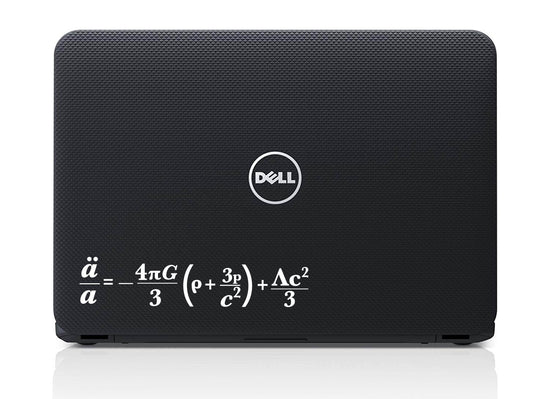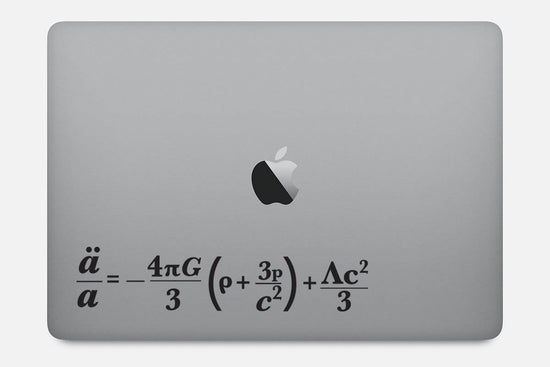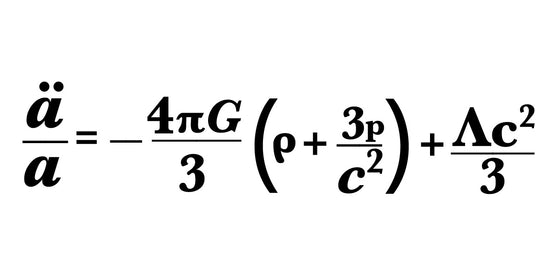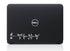Buttered Kat

# Friedmann Equation Decal Sticker

The Friedmann equation describes the expansion of space in homogeneous and isotropic models of the universe within the context of general relativity.
Where the ratio a:/a= H ( the Hubble constant),G, Λ, and c are universal constants (G is Newton's gravitational constant, Λ is the cosmological constant, and c is the speed of light in vacuum). k is constant throughout a particular solution, but may vary from one solution to another. a, H, ρ, and p are functions of time. ρ, and p are the density and pressure, respectively.

It can be applied to any smooth surface, comes in two sizes and seven colors. Contact us if you require custom size or color.

Proudly Made in the USA.
• chemistry
• Chemistry decal
• computer decal
• computer sticker
• Decal
• equation
• Physics
• Physics Decal
• science
Regular price
\$6.99
Sale price
\$6.99
Regular price

Couldn't load pickup availability

••••••••••••••••